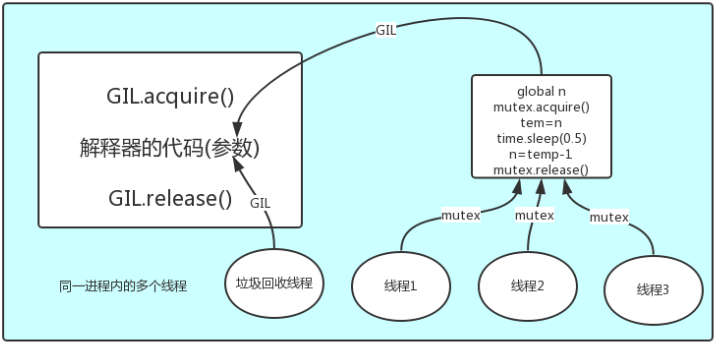#python-GIL、死锁递归锁及线程补充

0
0
01. 云栖社区>
2. 博客>
3. 正文

## 一、GIL介绍

GIL全称 Global Interpreter Lock ，中文解释为全局解释器锁。它并不是Python的特性，而是在实现python的主流Cpython解释器时所引入的一个概念，GIL本质上就是一把互斥锁，将并发运行变成串行，以此来控制同一时间内共享数据只能被一个任务所修改，从而保证数据的安全性。

```#1 所有数据都是共享的，这其中，代码作为一种数据也是被所有线程共享的（test.py的所有代码以及Cpython解释器的所有代码）

#2 所有线程的任务，都需要将任务的代码当做参数传给解释器的代码去执行，即所有的线程要想运行自己的任务，首先需要解决的是能够访问到解释器的代码。```## 二、GIL与Lock的区别

GIL保护的是解释器级别的数据，但是用户自己的数据需要自己加锁处理。```from threading import Thread,Lock
import time

mutex=Lock()
n=100
global n
with mutex:
temp=n
time.sleep(0.1)
n=temp-1

if __name__ == '__main__':
l=[]
for i in range(100):
l.append(t)
t.start()

for t in l:
t.join()
print(n)```
test

## 三、GIL与多线程

cpu是用来做计算的，多核，意味多个cpu去完成计算功能，提升计算性能，但是cpu一旦遇到 I/O操作，那么多核对I/O就没有什么帮助了。```#计算操作
from multiprocessing import Process
import os,time

def work():
res=0
for i in range(100000000):
res+=i

if __name__ == '__main__':
print(os.cpu_count())

p_l =[]
start = time.time()
for i in range(4):
p = Process(target=work)#5.057471036911011
p.start()
p_l.append(p)

for j in p_l:
j.join()
print('time is %s'%(time.time()-start))``````#io操作
from multiprocessing import Process
import time

def work():
time.sleep(4)

if __name__ == '__main__':
p_l = []
start = time.time()
for i in range(4):
p = Process(target=work)#4.281008243560791
p_l.append(p)
p.start()
for j in p_l:
j.join()
print(p_l)
print('time is %s' % (time.time() - start))```
I/O操作

多线程用于I/O密集型，如socket，爬虫，web等

多进程用于计算密集型，如金融分析等。

## 四、死锁与递归锁```from threading import Thread,Lock
import time
mutexA=Lock()
mutexB=Lock()

def run(self):
self.func1()
self.func2()
def func1(self):
mutexA.acquire()
print('\033[41m%s 拿到A锁\033[0m' %self.name)

mutexB.acquire()
print('\033[42m%s 拿到B锁\033[0m' %self.name)
mutexB.release()

mutexA.release()

def func2(self):
mutexB.acquire()
print('\033[43m%s 拿到B锁\033[0m' %self.name)
time.sleep(2)

mutexA.acquire()
print('\033[44m%s 拿到A锁\033[0m' %self.name)
mutexA.release()

mutexB.release()

if __name__ == '__main__':
for i in range(10):
t.start()

'''

'''```
test```from threading import Thread,Lock,RLock
import time

mutexB=mutexA=RLock()
#一个线程拿到锁，counter加1,该线程内又碰到加锁的情况，则counter继续加1，这期间所有其他线程都只能等待，等待该线程释放所有锁，即counter递减到0为止

def run(self):
self.f1()
self.f2()

def f1(self):
mutexA.acquire()
print('%s 抢到A锁' %self.name)
mutexB.acquire()
print('%s 抢到B锁' %self.name)
mutexB.release()
mutexA.release()

def f2(self):
mutexB.acquire()
print('%s 抢到了B锁' %self.name)
time.sleep(2)
mutexA.acquire()
print('%s 抢到了A锁' %self.name)
mutexA.release()
mutexB.release()

if __name__ == '__main__':
for i in range(100):
t.start()```
test

## 五、信号量Semaphore

Semaphore管理一个内置的计数器，```from threading import Thread,Semaphore
import time,random
sm=Semaphore(5)#最大连接数为5

sm.acquire()
print('%s 正在上厕所' %name)
time.sleep(random.randint(1,3))
sm.release()

if __name__ == '__main__':
for i in range(20):
t.start()```
test

## 六、Event```from threading import Thread,Event
import time

event=Event()

def light():
print('红灯正亮着')
time.sleep(3)
event.set() #绿灯亮

def car(name):
print('车%s正在等绿灯' %name)
event.wait() #等灯绿
print('车%s通行' %name)

if __name__ == '__main__':
# 红绿灯
t1.start()
# 车
for i in range(10):
t.start()```
test

## 七、queue补充

queue.LifoQueue() 后进先出---->堆栈```q=queue.LifoQueue(3)
q.put(1)
q.put(2)
q.put(3)
print(q.get())#3
print(q.get())#2
print(q.get())#1```
queue.LifoQueue

queue.PriorityQueue() 设置优先级别，数字越小，优先级别越高```q=queue.PriorityQueue(3) #优先级,优先级用数字表示,数字越小优先级越高
q.put((10,'a'))
q.put((-1,'b'))
q.put((100,'c'))
print(q.get())#(-1, 'b')
print(q.get())#(10, 'a')
print(q.get())#(100, 'c')```
PriorityQueue

+ 关注